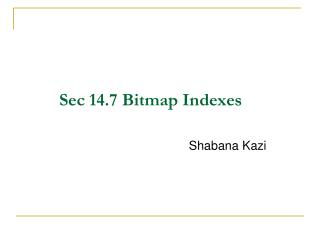Download PresentationSec 14.7 Bitmap Indexes

# Sec 14.7 Bitmap Indexes - PowerPoint PPT PresentationDownload Presentation## Sec 14.7 Bitmap Indexes

- - - - - - - - - - - - - - - - - - - - - - - - - - - E N D - - - - - - - - - - - - - - - - - - - - - - - - - - -
##### Presentation Transcript

1. Sec 14.7 Bitmap Indexes Shabana Kazi

2. Introduction A bitmap index is a special kind of index that stores the bulk of its data as bit arrays (commonly called "bitmaps"). A bitmap index for a field F is a collection of bit-vectors of length n. The vector for value v has 1 in position iif the ith record has v in field F, and it has 0 there if not. It answers most queries by performing bitwise logical operations on these bitmaps.

3. Example 1 Suppose a file consists of records with two fields, F and G, of type integer and string, respectively. The current file has six records, numbered 1 through 6, with the following values in order:

4. Example 1(contd…) A bitmap index for the first field, F, would have three bit-vectors, each of length 6 as shown in the table. In each case, the 1's indicate in which records the corresponding string appears.

5. Example (contd…) A bitmap index for the first field, G, would have three bit-vectors, each of length 6 as shown in the table. In each case, the 1's indicate in which records the corresponding string appears.

6. Motivation for Bitmap Indexes: Bitmap indexes can help answer range queries. Given is the data of a jewelry stores. The attributes considered are age and salary.

7. Motivation for Bitmap Indexes (contd…) A bitmap index for the first field Age, would have seven bit-vectors, each of length 12 as shown in the table. In each case, the 1's indicate in which records the corresponding string appears.

8. Motivation of Bitmap Indexes(contd…) A bitmap index for the second field Salary, would have ten bit-vectors, each of length 12 as shown in the table. In each case, the 1's indicate in which records the corresponding string appears.

9. Motivation of Bitmap Indexes(contd…) If we want to find the jewelry buyers with an age in the range 45-55 and a salary in the range 100-200. The bit-vectors for the age values in this range are found : 010000000100 and 001110000010, for 45 and 50, respectively. If we take their bitwise OR, we have a new bit-vector with 1 in position i if and only if the ith record has an age in the desired range. This bit-vector is 011110000110.

10. Motivation of Bitmap Indexes (contd…) • Next, we find the bit-vectors for the salaries between 100 and 200 thousand. • There are four, corresponding to salaries 100, 110, 120, and 140; their bitwise OR is 000111100000. • The last step is to take the bitwise AND of the two bit-vectors we calculated by OR. • That is: 011110000110 AND 000111100000 ----------------------------------- 000110000000

11. Motivation for Bitmap Indexes (contd…) We thus find that only the fourth and fifth records, which are (50,100) and (50,120), are in the desired range.

12. Compressed Bitmaps If there is a bitmap index on field F of a file with n records and there are m different values for field F. The number of bits in all the bit-vectors is mn. In our example, n= 12 and m=7

13. Compressed Bitmaps (contd..) • If the block size is 4096 bytes, then 32768 bits can fit in one block. Therefore the number of blocks needed is mn/32768. • If ‘m’ is large, then 1’s in a bit-vector will be rare. The probability that any can bit is 1 is 1/m. • If the 1’s are rare, then the bit-vectors can be encoded so that they take less than n bits on average. • A common approach is run-length encoding.

14. Compressed Bitmaps (contd..) • Example :If i= 13, then j = 4; that is, we need 4 bits in the binary representation of i. Thus. the encoding for i begins with 1110. We follow with iin binary, or 1101. Thus, the encoding for 13 is 11101101.

15. Compressed Bitmaps (contd..) • Example: Let us decode the sequence 11101101001011. Starting at the beginning. we find the first 0 at the 4th bit. So j = 4. The next 4bits are 1101.so we determine that the first integer is 13. We are now left with 11 001011 to decode. Since the first bit is 0: we know the next bit represents the next integer by itself. This integer is 0.

16. Compressed Bitmaps (contd..) • We find the first 0 in the second position, whereupon we conclude that the final two bits represent the last integer, 3. Our entire sequence of run-lengths is thus 13, 0, 3. From these numbers, we can reconstruct the actual bit-vector, 000000000000011000

17. Compressed Bitmaps (contd..) • Example: Convert the bit vectors for the three ages, 25 – 100000001000 30 – 000000010000 45 – 010000000100 For 25, the run-length sequence is (0,7) The bit vector is 00110111. For 30, run-length sequence is (7). The bit vector is 110111. For 45, the run-length sequence is (1,7), Bit vector is 01110111

18. Thank you